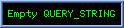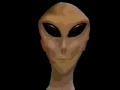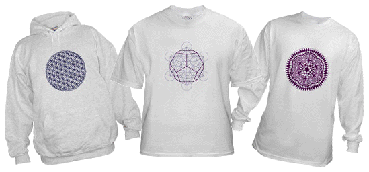Get a Metatron or Flower of Life T-shirt!
(click picture)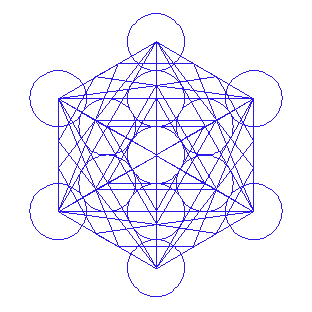Metatron's Cube.

3rd Dimension

# Sacred geometry, Metatron's cube and the platonic solidsBy IDTimeTraveller

The image you see above is the correct version of "Metatron's Cube", or the fruit of life with the 5 platonic solids. I made it with a 3D program and what you see are the actual edges of the platonic solids.

The dodecahedron and icosahedron actually have their "inner" corner points touching the star tetrahedron that fits in a circle that is =   1/Ø  x  the circle connecting the corners of the outer cube.
Where Ø ("phi")  =  1.618 033 988 749 594 ...

The way to figure out drawing this is by beginning with the normal flower of life "grid" (of 5 non-intersecting inner circles, or in this case: 7 intersecting full circles):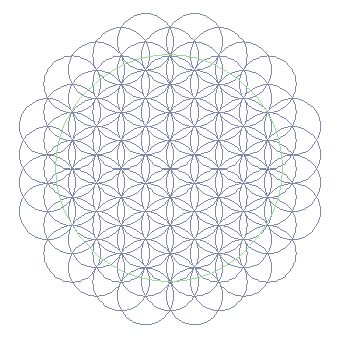Then draw a circle that is equal to   1/Ø  x  the outer circle.
For this, put the point of a compass on the middle of the vertical radius (in the centre of the second "flower" from the top along the vertical axis) and draw a circle from where the horizontal axis (through the middle of the circle) cuts the outer circle (see below);
The circle you can draw when you put one point of the compass in the centre of the grid, and draw a circle from where the other circle cuts the vertical axis (through the middle of the circle), is a circle Ø times smaller than the outer circle: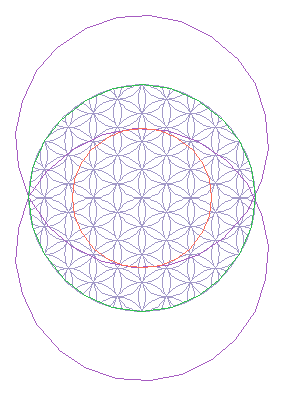After this, draw the appropriate startetrahedron(s) and you have found all the points necessary to draw the other platonic solids.

Here are all the platonic solids the way they fit in Metatron's cube:

Tetrahedron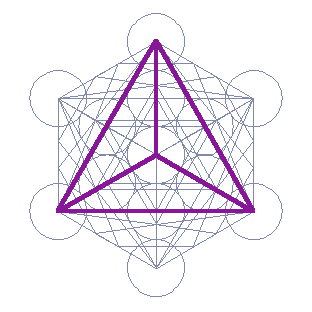Star tetrahedron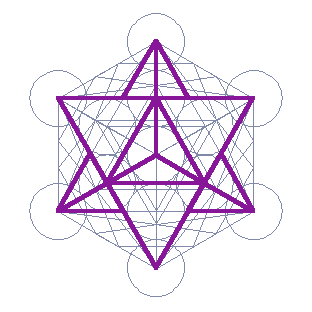Cube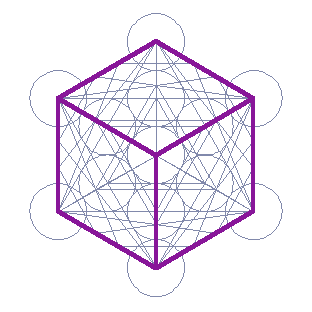Octahedron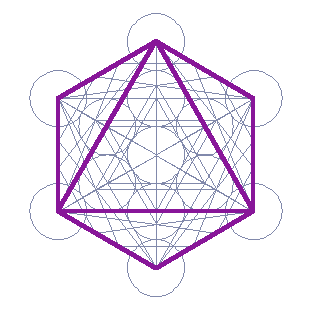Dodecahedron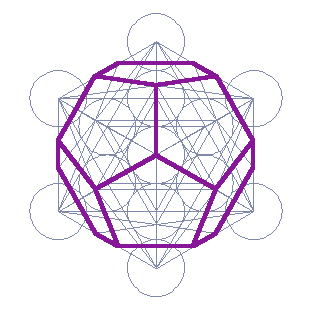Icosahedron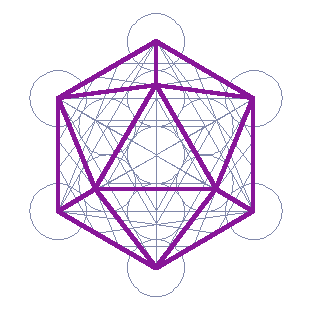All platonic solids

Not all solids can be (fully) seen, as some lines are hidden behind others.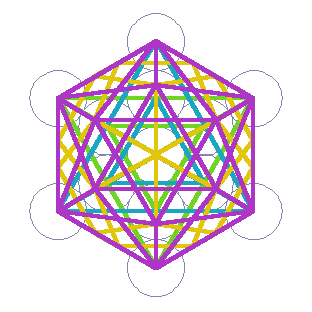All solids as in metatron's cube, seen from side

Here are all the solids of Metatron's cube. This is what you see in Metatron's cube from the top, but here it is seen from the (right) side.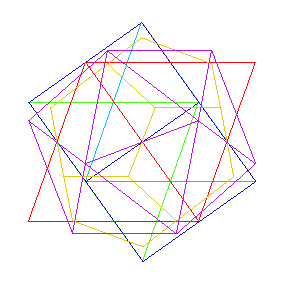Classic representation of dodecahdron in tetrahedron

This is a correct version of a dodecahdron in a startetrahedron. It's a classic representation that is commonly done in a way that is incorrect.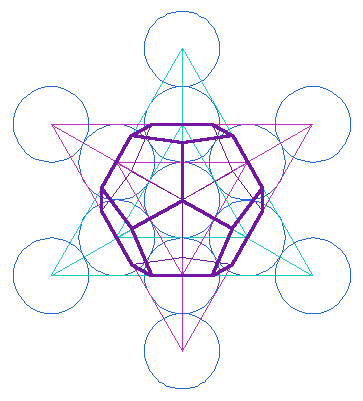click for a larger image

The corners of the dodecahedron are found by drawing lines from the corners of the big startetrahedron to those of a startetrahedron that is Ø times smaller. .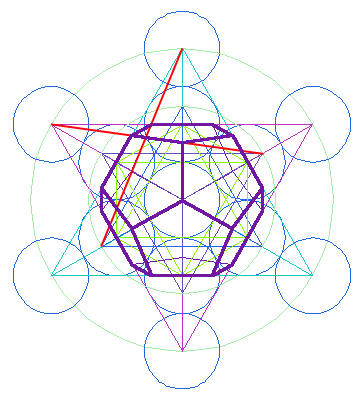click for a larger imageGet a Metatron or Flower of Life T-shirt!
(click picture)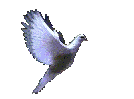Total amount of visits to this website: